# 2. Probability.

A property of a physical, biological or social system can generally assume different quantitative or qualitative values.

For example, the sex of a human being can be male or female; the stage of its life can be the childhood, the adolescence, the maturity or the oldness. Sex and stage of life are qualitative properties. Moreover a human being has age and height, both usually expressed by numbers. Age and height are quantitative properties.

The set, that can be discrete or continuous, of all the values that can be assumed by one or more properties of a system is the sample space or, more simply, the sample, S.

Every subset Ex of S is said an event.

A real function p(Ex) such that, given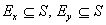,

•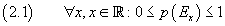•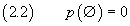•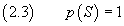•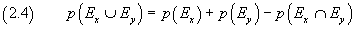•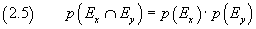is said probability function.

For example, the sample space of the outcomes of a roll of a dice is S={1,2,3,4,5,6}.

The events are subset as E1 = {1}, E2 = {2}, E3 = {3}, E4 = {4}, E5 = {5}, E6 = {6}, E12 = {1,2}, E246 = {2,4,6}, etc.

The equality (2.4) gives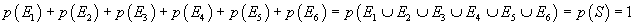so, if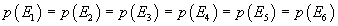, we have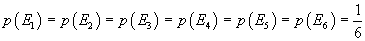We have also

•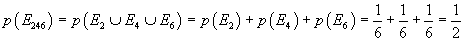•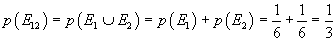•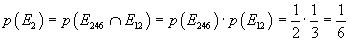If a sample space S is the cartesian product of two other sample spaces Sx and Sy and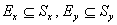, the symbol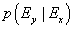(the probability of Ey given Ex) represents the probability of the event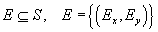. E is a compound event.

If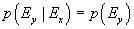, the events Ex and Ey are said mutually independent, or, more simply, independent; otherwise they are dependent.

If, for example, a box contains three balls, one red, one green and one blue and we draw a ball at random, the probability p(R) to draw a red ball is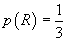.

If we do two consecutive extractions Ex and Ey, we have a compound event that is different depending on whether after the first extraction of a ball we put or not the ball in the box.

In the first case the sample space is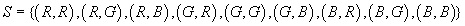.
There are 3 cases out of 9 in which the second ball is red, so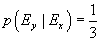. Then in this case the probability that the second ball is red is equal to the probability p(Ey)=p(R) of drawing a red ball in a single extraction: the events Ex and Ey are independent.

In the second case the sample space is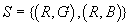and, as it is obvious, the second ball cannot be red: p(Ey|Ex)=0≠p(Ey).
The events Ex and Ey are dependent.

In general, if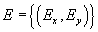is a compound event,

•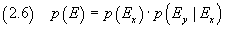In particular:

• if Ex and Ey are independent,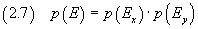• if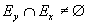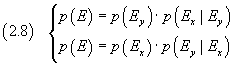The birthday problem

What is the probability that in a set of n randomly chosen people, two of them have the same birthday?

Let be p(n) the requested probability. The problem is easier if we first calculate the opposite probability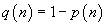. For simplicity's sake we do not consider the leap years and we assume that all dates of birth have equal probability.

• If there are n=2 people, the probability that the second was born on a different day from the first is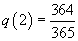• If there are n=3 people, the probability that all were born on different days is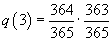• If there are n=4 people, the probability that all were born on different days is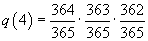• In general, by induction: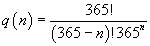• Finally: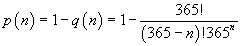For example, the probability that out of 80 people, there are 2 with the same birthday is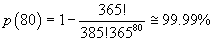: it is virtually certain that two people have the same birthday.

We could achieve the same result by using combinatorial methods. In fact, q(n) is given by the ratio between the combinations C365,n (that is all the set of n birthdays) and the permutations with repetition P365,n, noting that the permutations of the same elements are indistinguishable and should be counted only once.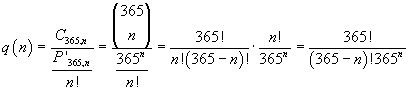Bernoulli trials

Let S be the sample space of the outcomes of an experiment andtwo events such that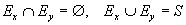.

If e is an outcome of the experiment, what is the probability that, in n repetitions of the same experiment,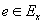k times?

We assume that the outcomes of the experiments are independent of each other and, for simplicity, we let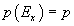so we can write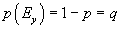.
q is said the opposite probability with respect to p.

The probability thatin k experiments and that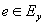in the remaining n-k experiments is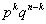.

Since this combination can have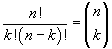permutations, the requested probability is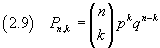Examples.

• If we roll a dice ten times, what is the probability of getting 1 two times?

The probability of getting 1 in a single throw of a dice is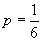, so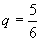. From (2.9) we have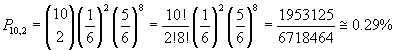• What is the probability of getting 1 at least once?

We may calculate the probability of not ever get 1: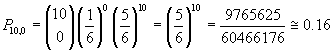and then calculate the opposite probability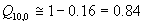Bayes' theorem

Let S be the sample space of an experiment and Ek (k from 1 to n) events of S such that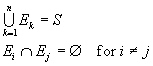Given any event E of S, we have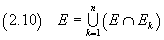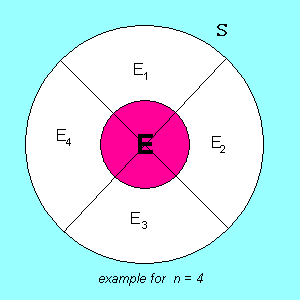From the equality (2.4) we get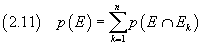and from the equality (2.5)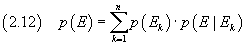The equality (2.12) is one way to express the Bayes' theorem.

Example.

In a school there are 600 girls and 400 boys. 40% of girls and 30% of kids have good grades in math. What is the probability that a student of that school has good grades in math?

Let be p(E) the requested probability, p(E1) the probability that a student is a girl, p(E2) the probability that a student is a boy, p(E|E1) the probability that a girls has good grades in math and p(E|E2) the same probability for a boy.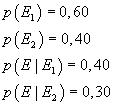From (2.12) we get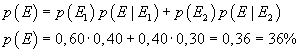More elementary:

• 600·40% = 240 girls have good grades;
• 400·30% = 120 boys have good grades;
• students with good grades are 240+120 = 360 out of 1000;
• the requested probability is 360/1000 = 36%.

From the equality (2.8) we get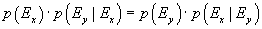If, in this equality, we let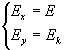we get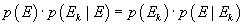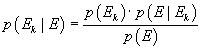and finally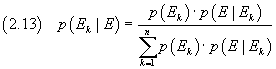The equality (2.13) is another way to express the Bayes' theorem.

In the previous example, what is the probability that a student with good grades is a girl?

From the equality (2.13) we get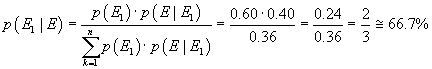More elementary:

• 240 girls have good grades;
• 120 boys have good grades;
• students with good grades are 360;
• girls are 240 out of 360;
• the requested probability is 240/360 = 66.7%.